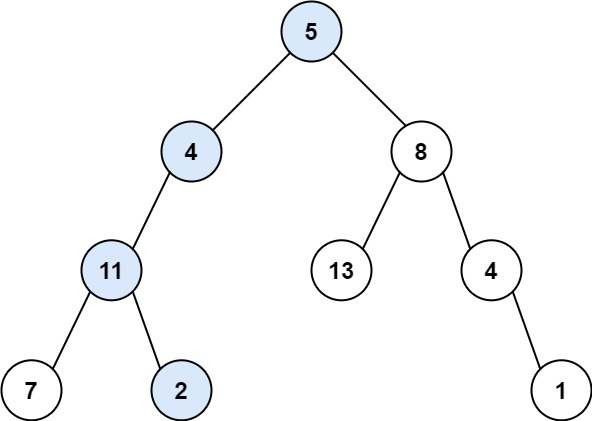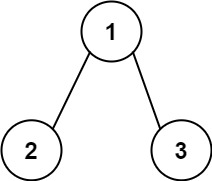# Path Sum

## 1: U-nderstand

Understand what the interviewer is asking for by using test cases and questions about the problem.

• Established a set (2-3) of test cases to verify their own solution later.
• Established a set (1-2) of edge cases to verify their solution handles complexities.
• Have fully understood the problem and have no clarifying questions.
• Have you verified any Time/Space Constraints for this problem?
• Can the input tree be Null?
• Yes, it can be. In that case there are no root-to-leaf paths, correct?
• Can a root-to-leaf path be the root itself, if it has no children?
• Yes, that can occur.
``````HAPPY CASE
Input: root = [5,4,8,11,null,13,4,7,2,null,null,null,1], targetSum = 22
Output: true
Explanation: The root-to-leaf path with the target sum is shown.````````````Input: root = [1,2,3], targetSum = 5
Output: false
Explanation: There two root-to-leaf paths in the tree:
(1 --> 2): The sum is 3.
(1 --> 3): The sum is 4.
There is no root-to-leaf path with sum = 5.````````````EDGE CASE
Input: root = [], targetSum = 0
Output: false
Explanation: Since the tree is empty, there are no root-to-leaf paths.``````

## 2: M-atch

Match what this problem looks like to known categories of problems, e.g. Linked List or Dynamic Programming, and strategies or patterns in those categories.

If you are dealing with Binary Trees some common techniques you can employ to help you solve the problem:

• Think about appropriate Tree Traversal: Pre-Order, In-Order, Post-Order, Level-Order
• Choosing a specific tree traversal that follows a general root-to-leaf path should help us identify all of the possible routes.
• Store nodes within a HashMap to refer to later
• We don’t have a specific way of referring to previous nodes in a path that could be used in a HashMap. So, a HashMap would not help us as much in this context.
• Using Binary Search to find an element
• We are not working with a Binary Search Tree.
• Applying a level-order traversal with a queue
• Using this approach may complicate our code

## 3: P-lan

Plan the solution with appropriate visualizations and pseudocode.

General Idea: Lets build the sum as we progress through the nodes. We will remove values from our targetSum as we progress. Upon reaching a leaf node, check if value is equal to targetSum.

``````1. Set basecase to root is None, return false
2. Upon reaching a leaf node, check if value is equal to targetSum
3. Recursively proceed to next node and remove value from targetSum ``````

⚠️ Common Mistakes

• Choosing the wrong traversal type
• Try to walk through the problem by hand and see the order in which you are processing the nodes. This will clue you into the type of traversal necessary
• Suppose you choose post order traversal, we would go left - right - check. Missing root values
• Suppose you choose in order traversal, we would go left - check - right. Missing root values

## 4: I-mplement

Implement the code to solve the algorithm.

``````# Definition for a binary tree node.
# class TreeNode:
#     def __init__(self, val=0, left=None, right=None):
#         self.val = val
#         self.left = left
#         self.right = right
class Solution:
def hasPathSum(self, root: Optional[TreeNode], targetSum: int) -> bool:
# Set basecase to root is None, return false
if not root:
return False

# Upon reaching a leaf node, check if value is equal to targetSum
if not root.left and not root.right:
return targetSum == root.val

# Recursively proceed to next node and remove value from targetSum
return self.hasPathSum(root.left, targetSum - root.val) or self.hasPathSum(root.right, targetSum - root.val)``````
``````class Solution {
public boolean hasPathSum(TreeNode root, int sum) {
// Set basecase to root is None, return false
if (root == null) return false;

// Upon reaching a leaf node, check if value is equal to targetSum
if (root.left == null && root.right == null && root.val == sum) return true;

// Recursively proceed to next node and remove value from targetSum
return hasPathSum(root.left, sum - root.val) || hasPathSum(root.right, sum - root.val);
}
}``````

## 5: R-eview

Review the code by running specific example(s) and recording values (watchlist) of your code's variables along the way.

• Trace through your code with an input to check for the expected output
• Catch possible edge cases and off-by-one errors

## 6: E-valuate

Evaluate the performance of your algorithm and state any strong/weak or future potential work.

Assume `N` represents the number nodes in tree

• Time Complexity: `O(N)` because we need to visit each node in binary tree.
• Space Complexity: `O(1)` if we do not count the recursion stack. The recursion stack will cost us `O(N)` if we have a linked list like tree. `O(logN)` in the average case.